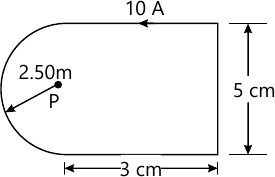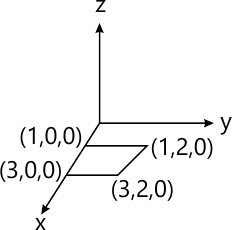Total marks: --
Total time: --
INSTRUCTIONS
(1) Assume appropriate data and state your reasons
(2) Marks are given to the right of every question
(3) Draw neat diagrams wherever necessary

1 (a) State and prove Gauss law.
4 M
1 (b) Find the volume charge density at (4m, 45', 60'). If the electric flux density is given by, $\overrightarrow {D} = (r \widehat {a_r}+\sin\theta \widehat{a_s} + \sin \theta \cos \phi \widehat_{a_\phi}) C/m^2.$
6 M
1 (c) Given $\overrightarrow {D} = \dfrac {10r^3} {4} \widehat{a_r}$ in cylindrical co-ordinates, evaluate both sides of the divergence theorem for the volume enclosed by the cylinder with r=2 m, z=0 to 10 m.
10 M

2 (a) With usual notations, prove point form of continuity equation, $\nabla.J = \dfrac {\partial \rho_v} {\partial t} .$
5 M
2 (b) Find the amount of energy required to move a 6 coulomb of point charge from the origin to P(3, 1, -1)m in the field $\overrightarrow{E} = (2x, \widehat{a_x} - 3y^2 \widehat{a_y} + 4a_x )$ V/m along the straight line path, x=-3z, y=x+2z.
5 M
2 (c) A parallel plate capacitor is filled with a dielectric of 0.03 power factor and εr=10. The plates have an area of 250 mm2 and the distance between them is 10 mm. If 5000 V (RMS) at 1 Mhz is applied to the capacitor find the power dissipated as heat.
10 M

3 (a) Find V and the volume charge density in free space, if $V= \dfrac { 2 \cos \phi} {r^2} \ at \ P(0.5, 45^{\circ}, 60^{\circ}).$
7 M
3 (b) Find the electric field at P(3, 1, 2) for the field of two radial conducting planes V=50 V at ϕ=10° and V=20 V at ϕ=30°.
8 M
3 (c) State and prove uniqueness theorem.
5 M

4 (a) State and prove Ampere's law.
4 M
4 (b) Calculate the magnetic field intensity at point P due to 10 A current flowing in the anticlockwise direction in the metallic block shown in Fig. Q4 (b).6 M
4 (c) Verify strokes theorem for a field having $\overrightarrow {H} = 2\rho^2 (\tau +1) \sin \phi \widehat{a_\phi}$ for the portion of a cylindrical surface defined by $\rho = 2, \ \dfrac {\pi}{4} \le \phi \le \dfrac {\pi} {2}, \ 1\le \tau \le 1.5$ and for its perimeter.
10 M

5 (a) What is Lorentz force equation?
2 M
5 (b) A square loop carrying 2 mA current is placed in the field of an infinite element carrying current of 15 A as shown in Fig Q5 (b). Find the force exerted on the loop.8 M
5 (c) Two homogeneous, linear, isotropic material have interface at X=0, in which there is a surface current $$K=200 \widehat{a_z}$$ A/m. In region 2 for X>0, find i) H2 ii) |B1| iii) |B2|
iv) α1 v) α2. \[ if \ H_1 = (150 \widehat{a_x} - 400 \widehat{a_y} + 250\widehat{a_z}) A/m.
10 M

6 (a) State Maxwell's equations for a good conductor and for perfect dielectrics.
8 M
6 (b) Define phase velocity, wavelength and propagation constant.
6 M
6 (c) A uniform plane wave traveling in +z direction in air has $$H=20\widehat{a_y}$$ <\span> A/m the frequency of the signal is \( \dfrac {1}{\pi} \times 10^9 Hz. Find λ, T and E.
6 M

7 (a) Derive the expression for α, β, γ and V for low loss dielectric.
6 M
7 (b) For a uniform plane wave. Ey=10.4 e(-jβτ+2π×105t) V/m. Find
i) The direction of propagation.
ii) Phase constant β
iii) Expression for H.
5 M
7 (c) A material is characterized by εr=2.5, μr=1 and σ = 4×10-5℧/m at f=1 Mhz. Determine the value of the loss tangent, attenuation constant and phase constant.
9 M

Write explanatory notes on:
8 (a) Standing wave ratio.
8 M
8 (b) Poynting vector.
12 M

More question papers from Field Theory